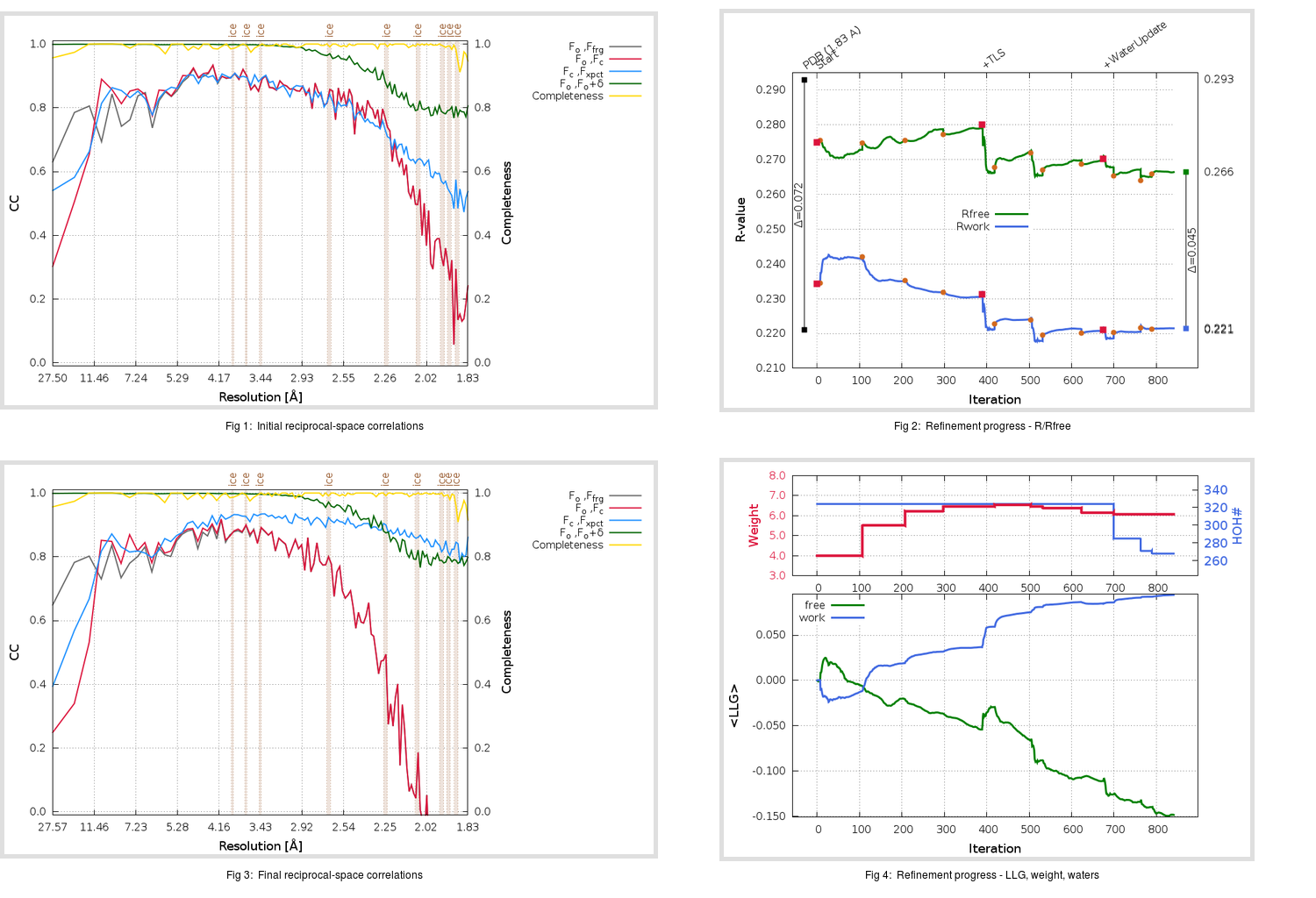Content:

```    Diffraction limits & principal axes of ellipsoid fitted to diffraction cut-off surface:
1.816         0.8805   0.0000   0.4741       0.992 a* + 0.126 c*
1.770         0.0000   1.0000   0.0000       b*
1.754        -0.4741   0.0000   0.8805      -0.784 a* + 0.620 c*
```

## Deposited

` `
 Date deposited Date data collection Resolution R, Rfree 20200303 20200227 1.83 0.2170 0.2930

Molprobity (CCP4 7.0 version) summary:

```Ramachandran outliers =   0.66 %
favored =  96.03 %
Rotamer outliers      =   3.41 %
C-beta deviations     =     0
Clashscore            =   5.93
RMS(bonds)            =   0.0142
RMS(angles)           =   1.86
MolProbity score      =   2.00
Resolution            =   1.83
R-work                =   0.2170
R-free                =   0.2930
```

```Number of waters      =   324

<B> (all atoms) =   38.22 ( sd =   11.36 ) for       2725 non-hydrogen atoms
<B>   (protein) =   37.30 ( sd =    9.61 ) for       2373 non-hydrogen atoms
<B>     (water) =   46.17 ( sd =   20.54 ) for        324 non-hydrogen atoms
<B>    (others) =   69.08 ( sd =   53.39 ) for         28 non-hydrogen atoms

B min/max       (all non-hydrogen atoms) =   19.11 /  196.02
B min/max   (protein non-hydrogen atoms) =   21.24 /   90.45
B min/max     (water non-hydrogen atoms) =   21.85 /  196.02
B min/max     (other non-hydrogen atoms) =   19.11 /  135.23
```

## BUSTER (re-)refinement

` `

Molprobity (CCP4 7.0 version) summary:

```Ramachandran outliers =   0.66 %
favored =  97.02 %
Rotamer outliers      =   1.89 %
C-beta deviations     =     0
Clashscore            =   2.54
RMS(bonds)            =   0.0118
RMS(angles)           =   1.57
MolProbity score      =   1.42
Resolution            =   1.83
R-work                =   0.2214
R-free                =   0.2664
```

```Number of waters      =   268

<B> (all atoms) =   38.80 ( sd =    8.72 ) for       2669 non-hydrogen atoms
<B>   (protein) =   38.93 ( sd =    7.28 ) for       2373 non-hydrogen atoms
<B>     (water) =   36.27 ( sd =   17.04 ) for        268 non-hydrogen atoms
<B>    (others) =   43.27 ( sd =   22.68 ) for         28 non-hydrogen atoms

B min/max       (all non-hydrogen atoms) =   21.40 /   77.11
B min/max   (protein non-hydrogen atoms) =   26.02 /   77.11
B min/max     (water non-hydrogen atoms) =   21.40 /   70.09
B min/max     (other non-hydrogen atoms) =   26.35 /   67.45
```

Refinement progression:Results:

` `
 File Remark 5R84_aB_refine.01_03_refine.pdb.gz exact refinement commands are in header 5R84_aB_refine.01_03_refine.mtz.gz including original deposited data and several re-refinement map coefficients 5R84_aB_refine.01_03_BUSTER_model.cif.gz including any non-standard compound restraints 5R84_aB_refine.01_03_BUSTER_refln.cif.gz